# A rectangular

A rectangular garden 40 m long and 30 m wide is to be fenced with fence posts at each corner. All the other posts will be 5 meters a part. How many posts will be needed to fence in the garden.

n =  28

### Step-by-step explanation:Did you find an error or inaccuracy? Feel free to write us. Thank you!#### You need to know the following knowledge to solve this word math problem:

We encourage you to watch this tutorial video on this math problem:

## Related math problems and questions:

• GardenRectangular garden 31 meters 40 centimeters long and 20m30cm wide adjacent with the shorter side with another (fenced) garden and on one longest side is 1 meter wide gate. How many meters of fence is needed to buy? (Estimate, calculate, verify a test calc
• Triangular landJana has a rectangular garden measuring 30 meters by 72 meters that she wants to split diagonally from corner to corner using a fence. How long does her fence need to be?
• Fence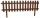How many meters of fencing it is required to purchase if our garden is rectangular measuring 20 m and 180 dm.
• Garden fence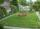The garden has the shape of a rectangular triangle with an area of 96 square meters and a 16 m long one leg. How many meters of the fence need to be fenced?
• Decorative fenceThe rectangular garden is 57 m long and 42 m wide. Calculate how much m² it will reduce if it encloses with a 60 cm wide decorative fence.
• Fence of the garden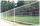The garden has dimensions of 5m and 400cm. How many meters meshes are needed for fencing the plot?
• PlaygroundThe rectangular playground is fenced with 38 m long netting. Its width is 7 m. Calculate its length.
• Fence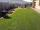The square garden has an area of 537 m2. How many meters is netting necessary to fence the garden?
• Rectangular gardenRectangular garden has a length of 48.7 m, a width of 6.3 meters shorter than the length. How much mesh should be bought for its fencing if the gate is 2.9 m long and the gate 1.1 m? What is the area of the garden?
• Garden plotCalculate how many meters of fence need to fence the square garden with length and width of 12 meters.
• The gardenThe garden has the shape of a rectangular trapezium. The bases have lengths of 27 meters and 36 meters, the trapezoid's height is 12 meters. Calculate how much fence will cost this garden if one meter costs 1.5 €?
• GardenThe rectangular garden has dimensions of 27 m and 30 m. Peter and Katka split it in a ratio of 4:5. How many square meters did Katkin measure part of the garden?
• Wire fenceThe wire fence around the garden is 160 m long. One side of the garden is three times longer than the other. How many meters do the individual sides of the garden measure?
• Rectangular garden 2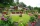A farmer bought 600 m of wire for the fence. He wants to use it to besiege a rectangular garden with a surface of 16875 m2. Calculate the size of the garden.
• Rectangular gardenThe perimeter of Peter's rectangular garden is 98 meters. The width of the garden is 60% shorter than its length. Find the dimensions of the rectangular garden in meters. Find the garden area in square meters.
• The rawThe raw data presented here are the scores (out of 100 marks) of a market survey regarding the acceptability of new product launched by a company for random sample of 50 respondents: 40 45 41 45 45 30 30 8 48 25 26 9 23 24 26 29 8 40 41 42 39 35 18 25 35
• GardensThe garden has the square shape with circumference 124 m. Divide it into two rectangular gardens, one should circumference 10 meters more than the second. What size will have a gardens?